The STATESPACE Procedure

OUTMODEL= Data Set

The OUTMODEL= data set contains the estimates of the
andmatrices and their standard errors, the names of the components of the state vector, and the estimates of the innovation covariance matrix. The variables contained in the OUTMODEL= data set are as follows:

• the BY variables

• STATEVEC, a character variable that contains the name of the component of the state vector corresponding to the observation. The STATEVEC variable has the value STD for standard deviations observations, which contain the standard errors for the estimates given in the preceding observation.

• F_j, numeric variables that contain the columns of thematrix. The variable F_j contains the jth column of. The number of F_j variables is equal to the value of the DIMMAX= option. If the model is of smaller dimension, the extraneous variables are set to missing.

• G_j, numeric variables that contain the columns of thematrix. The variable G_j contains the jth column of. The number of G_j variables is equal to r, the dimension of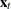given by the number of variables in the VAR statement.

• SIG_j, numeric variables that contain the columns of the innovation covariance matrix. The variable SIG_j contains the jth column of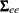. There are r variables SIG_j.

Table 28.3 shows an example of the OUTMODEL= data set, with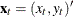,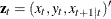, and DIMMAX=4. In Table 28.3,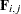and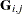are the i,jth elements ofandrespectively. Note that all elements for F_4 are missing becauseis a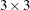matrix.

Table 28.3: Value in the OUTMODEL= Data Set

Obs

STATEVEC

F_1

F_2

F_3

F_4

G_1

G_2

SIG_1

SIG_2

1

X(T;T)

0

0

1

.

1

0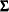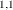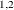2

STD

.

.

.

.

.

.

.

.

3

Y(T;T)

F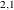F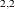F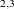.

0

14

STD

std Fstd Fstd F.

.

.

.

.

5

X(T+1;T)

F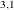F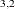F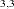.

GG.

.

6

STD

std Fstd Fstd F.

std Gstd G.

.